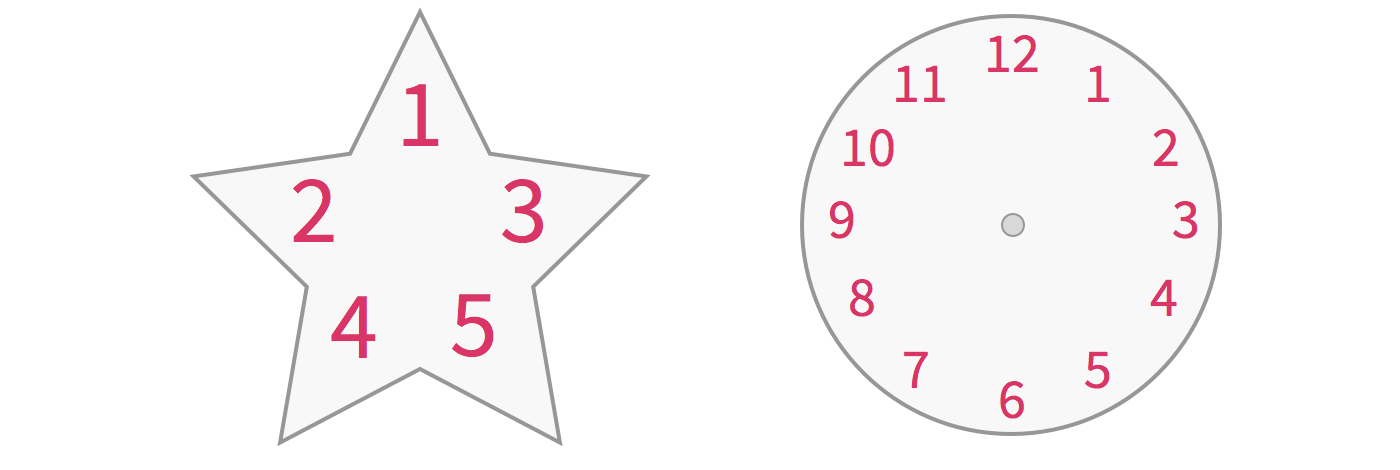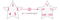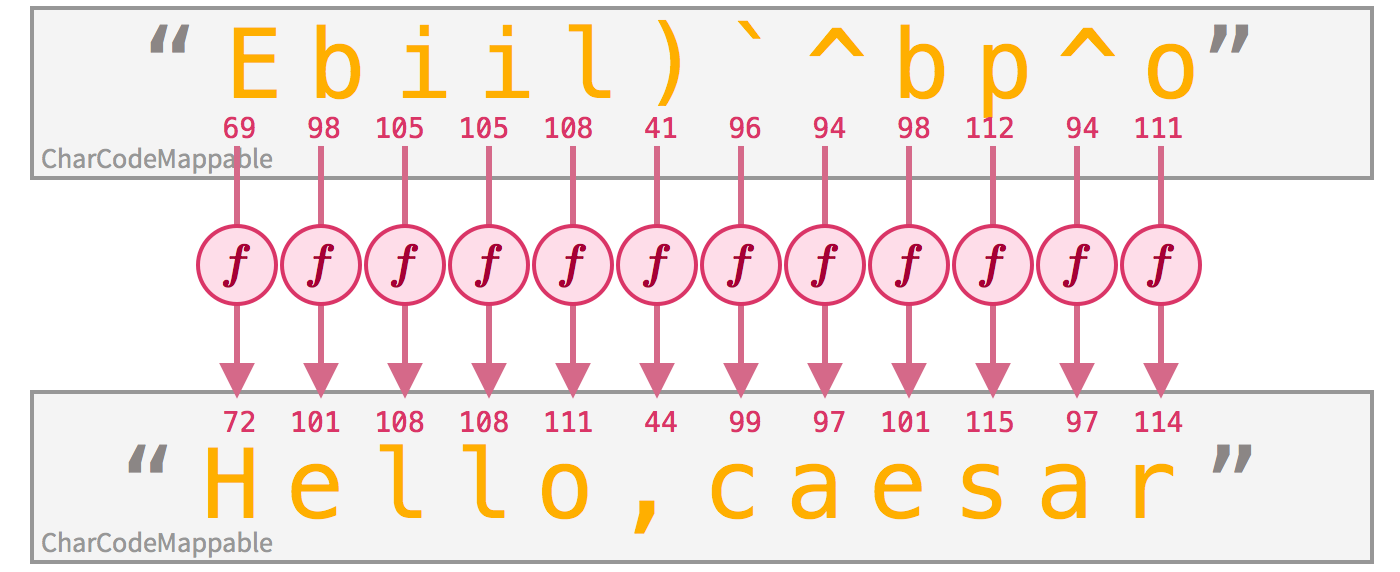# What is a functor?

This is my attempt at explaining about a functional programming concept called ‘functor’ in an easy-to-understand way. I’ll be explaining it in JavaScript.

I hope everyone reading this will be able to follow along. If not, please feel free to leave me some notes! :)

According to Haskell and the Fantasy Land specification, a functor is simply something that can be mapped over. In OOP-speak, we’d call it a ‘Mappable’ instead.

You probably have used some functors before (maybe without knowing that it is a functor). Some of you may say, “Hey, I know what can be mapped over. An array can be mapped over — you can map a function over an array! Look:”

`console.log([ 2, 4, 6 ].map(x => x + 3))// => [ 5, 7, 9 ]`

You’re right! This means an array is a functor! (At this point, if you don’t understand what map is, please watch this video first.)

In fact, we can create a functor out of (mostly) any value. Even single values, strings and ordinary objects. Even functions. We’ll see how in this article.

First, let’s define ‘something’: That ‘something’ is simply a set of values arranged in some shape (and under some constraints).The values are in red, and the shape is in gray.

An array is a set (or a collection) of values, because it’s a list of values (this should be pretty obvious to you).

An object is also a set of values, because it can represent a collection of values, identified by keys.

Here’s a Wrapper class, whose instance simply wraps a single value:

`class Wrapper {  constructor (value) {    this.value = value  }}`

What a stupid class. An instance of this Wrapper class is also a set of values. You have to think of it as a special kind of set that can hold only one value — no more, no less. That’s the constraint.

Technically, it’s called an algebra (wut?), but we won’t be using that term because it sounds too nerdy. We’ll stick with the word something.

Now, let’s define ‘that can be mapped over’: It means that you can take all the values inside it, then for each value, you derive something out of it (by calling a function). You then put these resulting values back into a new container of the same structure and shape.

Note that although the shape and structure must stay the same, the type may change. Your mapper function f needs not return the same type.Although the shape and structure are retained, the numbers are mapped into strings.

We’ll look at several examples to see how we can map functions over things.

# Array

This picture from the article Functors, Applicatives, And Monads In Pictures by Aditya Bhargava shows how an array can be mapped over. (That article, by the way, is really, really good.)

There are more rules to it, but let’s discuss it later.

In code, how do we map a function over an array? We call the .map(f) method and provide it a function.

This is how you should map a function over things in JavaScript, according to the Fantasy Land Specification.

Let’s try it with arrays.

`const addThree = (x) => x + 3const array = [ 2, 4, 6 ]const mappedArray = array.map(addThree)console.log(mappedArray)// => [ 5, 7, 9 ]`

Since an array is something that can be mapped over, it is a functor.

# Single value

Remember that Wrapper class that wraps a single value?

We can map a function over it by taking the wrapped value, apply some function, and put it in a new wrapper.

We’ll extend the Wrapper with .map(f) method. Now it’s a functor.

`class Wrapper {  constructor (value) {    this.value = value  }  map (f) {    return new Wrapper(f(this.value))  }}`

Let’s try it:

`const something = new Wrapper(39)console.log(something)// => { value: 39 }const mappedSomething = something.map(addThree)console.log(mappedSomething)// => { value: 42 }`

I hope you see now why instances of the Wrapper class is a functor.

# Object

We can think of plain objects in JavaScript as a set of values. Each value is identified by some key.

Now, for ordinary JavaScript objects, you can’t just map a function over it, because JavaScript objects usually don’t have a .map(f) method.

We need to create a wrapper/functor that lets you map each value inside that wrapped object. We’ll call it ValueMappable.

`class ValueMappable {  constructor (object) {    this.object = object  }  map (f) {    const mapped = { }    for (const key of Object.keys(this.object)) {      mapped[key] = f(this.object[key])    }    return new ValueMappable(mapped)  }}`

What is does is this: It takes each value inside the wrapped object, apply a function over it, and put it in a new wrapper that wraps an object of the same shape.

Let’s try it:

`const myData = { myAge: 22, friendAge: 21 }const myDataFunctor = new ValueMappable(myData)const threeYearsLaterFunctor = myDataFunctor.map(addThree)const threeYearsLater = threeYearsLaterFunctor.objectconsole.log(threeYearsLater)// => { myAge: 25, friendAge: 24 }`

# String

How about a String? We can also think of it as an ordered sequence of character codes*. This means it can be mapped over!This functor is quite special because the numbers are not being stored ‘physically.’ In this case, a string is a collection of characters, but the character codes that make up the string are represented as numbers on-the-fly.

Unfortunately, String does not provide a map method. So in JavaScript by default it’s not a functor.

(*) Also note that there is more than one way to represent a string as a set of values. You can think of it as a sequence of 16-bit character codes (charCode). You can also see it as a sequence of unicode code points (codePoint). Or maybe a sequence of UTF-8 encoded byte values.

So again, we need to create a wrapper that acts as a functor. (You can also extend String’s prototype, but I don’t think you’d want to do it.)

`class CharCodeMappable {  constructor (string) {    this.string = string  }  map (f) {    let string = this.string    let result = ''    for (let i = 0; i < string.length; i++) {      result += String.fromCharCode(        f(string.charCodeAt(i))      )    }    return new CharCodeMappable(result)  }}`

Let’s try it!

`const mySecretString = 'Ebiil)`^bp^o'const mySecretStringFunctor = new CharCodeMappable(mySecretString)const decodedStringFunctor = mySecretStringFunctor.map(addThree)const decodedString = decodedStringFunctor.stringconsole.log(decodedString)// => "Hello,caesar"`

Edit: I was wrong.
It turns out that String / CharCodeMappable is NOT a functor.
See this response for an explanation and discussion.

In short, a functor that holds values of type A, when mapped over with a function that takes a value of type A and returns a value of type B, the result must be a functor that holds values of type B.

This is not the case for the above example, because CharCodeMappable only (conceptually) holds number. You may .map(x => String(x)) over an Array of numbers, but not over a CharCodeMappable!

# Function

When I first learned that a function is in fact a functor (I learned it from Learn You a Haskell for Great Good!), it made me confused.

A function is a functor? A functorception?!?
What’s going on here?

Note that we’re talking about pure functions. How can we view a function as a ‘set of values?’

Let’s try by example. Here’s the square function:

`const square = (x) => x * x`

You can think of this function as an infinite set of all the numbers this function can produce. In this case, it’s a set of non-negative numbers.

For example, the value 4 is in this set, because you can retrieve it by calling square(2) or square(-2). It also holds value 100, because you can get it by calling square(10) or square(-10). NaN is also in this set.

But negative numbers are not in this set, because no matter what number you put into this function, you won’t get a negative number back.

Now, imagine that I took all the numbers inside this set, and add each number by 3 (thereby mapping that function/functor with addThree, another function). What do I get back?

You’ll get a set of numbers not less than 3:

And it’s possible to actually do that in code. We just don’t apply the mapping beforehand; we apply it on-the-fly:

`const squareMapped = (x) => addThree(x * x)`

Attentive readers will notice that mapping a function over another function is simply function composition:

For the sake of example, I’ll be very naughty and just implement Function.prototype.map:

`Function.prototype.map = function (f) {  const g = this  return function () {    return f(g.apply(this, arguments))  }}`

Let’s try it:

`const squareMapped = square.map(addThree)console.log(squareMapped(2))// => 7console.log(squareMapped(10))// => 103`

You see, it seems like I took every possible value that the square function can produce, and added it by three.

Now you see how a function can become a functor.

# Observer Pattern

The observer pattern is a popular design pattern in OOP world.

Basically, the subject is open for other objects (called observers) to subscribe. When some interesting event occurs, the subject notifies all the observing parties, passing along some data payload.

For instance, a subject may connect to a Twitter stream, and notifies the observers that has subscribed to it when someone you follow tweets.

`tweets.subscribe(function (tweet) {  console.log(`@\${tweet.user.screen_name}: \${tweet.text}`)})// (At 10 am:) @dtinth: Good morning!// (At 1 pm:)  @dtinth: Good afternoon!// (At 11 pm:) @dtinth: Good night!`

You can view a subject as a set of payloads that will be published by this subject over time. In above example, this is a set of tweets. You only get access to these tweets when the person actually tweets it.

Let’s say we’re only interested in the tweet’s length. We have this function that takes the length of the tweet.

`const lengthOfTweet = (tweet) => tweet.text.length`

Assuming this subject is a functor, we should be able to do this:

`tweets.map(lengthOfTweet).subscribe(function (length) {  console.log(`Someone posted a \${length}-character tweet!`)})// (At 10 am:) Someone posted a 13-character tweet!// (At 1 pm:)  Someone posted a 15-character tweet!// (At 11 pm:) Someone posted a 11-character tweet!`

This is one of the ideas behind functional reactive programming libraries such as RxJS and Bacon.js.

# The functor laws

Of course, the .map(f) method cannot just be some random function and then you’d call any object with the .map(f) method a functor!

There are rules on how .map(f) should behave, so that it can qualifies as a functor.

The identity law:
functor.map(x => x) ≡ functor

This means if you map over some functor with a function that simply returns the passed value (the identity function), the resulting functor should be equivalent to the original functor:

`console.log([ 0, 1, 2, 3 ].map(x => x))// =>       [ 0, 1, 2, 3 ]`

Now, let’s look at the second law.

The composition law:
functor.map(x => f(g(x))) ≡ functor.map(g).map(f)

Learn You a Haskell for Great Good! did a very good job at stating this law in plain English, so I’ll just quote them here.

“The second law says that composing two functions and then mapping the resulting function over a functor should be the same as first mapping one function over the functor and then mapping the other one.”

“If we can show that some type obeys both functor laws, we can rely on it having the same fundamental behaviors as other functors when it comes to mapping.”

Let’s say I have some data like this:

`const ceos = {  Apple:     { firstName: 'Tim', lastName: 'Cook' },  Microsoft: { firstName: 'Satya', lastName: 'Nadella' },  Google:    { firstName: 'Sundar', lastName: 'Pichai' }}`

I have a function that takes a person object, and returns the full name:

`const fullNameOfPerson = (person) => (  person.firstName + ' ' + person.lastName)`

I have another function that takes a name, and generates a greeting:

`const greetingForName = (name) => `Hello, \${name}!``

I also have a function that does both:

`const greetingForPerson = (person) => (  greetingForName(fullNameOfPerson(person)))`

First, I’m going to map over this data to obtain their full names. Then I’m going to map over the resulting object again to generate greetings.

`const greetings1 = (new ValueMappable(ceos)  .map(fullNameOfPerson)  .map(greetingForName)  .object)console.log(greetings1)// => { Apple:     'Hello, Tim Cook!',//      Microsoft: 'Hello, Satya Nadella!',//      Google:    'Hello, Sundar Pichai!' }`

Next, I’m going to map over this data again, but this time I’m going to do both at the same time.

`const greetings2 = (new ValueMappable(ceos)  .map(greetingForPerson)  .object)console.log(greetings2)// => { Apple:     'Hello, Tim Cook!',//      Microsoft: 'Hello, Satya Nadella!',//      Google:    'Hello, Sundar Pichai!' }`

This means you can freely compose and group .map(f) calls as you wish!

# Summary

We’ve learned that a functor represents a set of things (in some structure) that can be mapped over to obtain a set of new things under that same structure.

We use the .map(f) method to perform that mapping. An array is one popular functor.

Unfortunately, not many things in JavaScript provide a .map(f) method when it can somewhat be mapped over. To make a functor out of it, we either need to extend that type, or create a wrapper that acts as a functor.

Functors can be useful because we don’t have to care about its structure. We only care that we can map some function over it. Does that remind you of polymorphism?

Anyway, I hope you have a better understanding of functors and of mapping over something other than an array. Thanks for reading! :)

Update: Based on the responses, I’ve added more references.

# Appendix I: Other Definitions

As with many programming terms, different programmers tend to associate different meanings to the same term.

In the past, I’ve struggled through different definitions of Model-View-Controller pattern, where everyone interprets each term a bit differently. About two months ago, also I explored about different definitions of inheritance.

In this article, I talk about Functors in the way that is interpreted by Haskell, category theory, and the Fantasy Land specification. But I’ve also found several other definitions for functors:

• A post in Functional JavaScript Blog states that a functor is a function that, “given a value and a function, unwraps the values to get to its inner value(s), calls the given function with the inner value(s), wraps the returned values in a new structure, and returns the new structure. […] Also the returned structure need not be of the same type as the original value.” In this case, all the examples in “what’s NOT a functor” sequel is a valid functor.
• The Wikipedia page for Higher-order function states that a functor is just another synonym for higher-order function). In this case, all higher-order function is a functor.
• The Apache Commons Functor project states that “A functor is a function that can be manipulated as an object, or an object representing a single, generic function.”

It seems that the word functor is losing its original meaning.

# Appendix II: Sequels

(@dtinth) A software engineer and frontend enthusiast. A Christian. A JavaScript musician. A Ruby and Vim lover.

(@dtinth) A software engineer and frontend enthusiast. A Christian. A JavaScript musician. A Ruby and Vim lover.